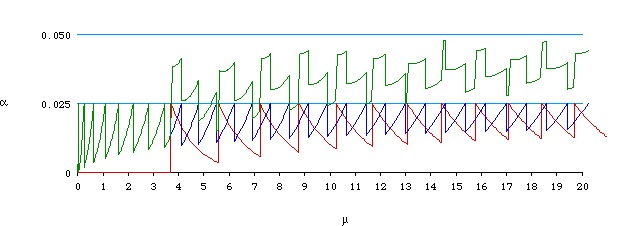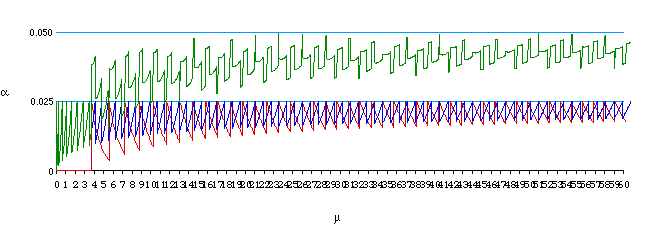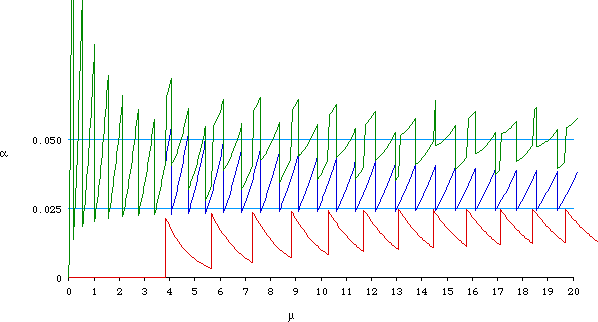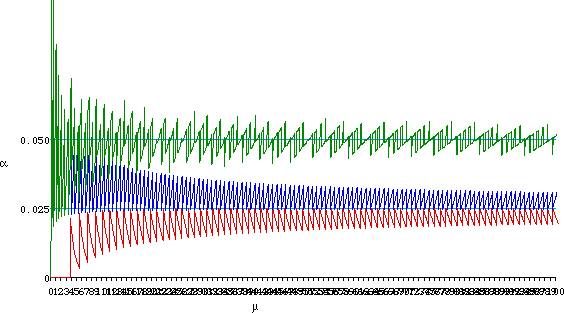## Confidence Intervals for the Mean of a Poisson Distribution

### "Exact" 95% Confidence Intervals

Let x be a single observation from a Poisson distribution with mean µ. Then "exact" 95% confidence limits for µ are given by the formula

( qchisq(0.025, 2*x)/2, qchisq(0.975, 2*(x+1))/2 )

These limits can be computed in S or taken from chi-square tables.

 x Lower Limit Upper Limit 0 0.0000 3.6889 1 0.0253 5.5716 2 0.2422 7.2247 3 0.6187 8.7673 4 1.0899 10.2416 5 1.6235 11.6683 6 2.2019 13.0595 ... ... ...

To compute the probability that the interval will miss the true value of µ, consider first the upper limit. Note that the upper limit is never less than 3.6889, so if µ < 3.6889 then the chance that the upper limit will miss the true value is zero. If 3.6889 < µ < 5.5716 the upper limit will miss the true value of µ if and only if x = 0 and the chance of this happening is computed as a left-tail probability of a Poisson distribution with mean µ. If 5.5716 < µ < 7.2247 the upper limit will miss the true value of µ if and only if x <= 1, and so on. In general, then, using the relationship between Poisson left tail probabilities and chi-square right tail areas, we find that if x' satisfies

qchisq(0.975, 2*(x'+1))/2 < µ < qchisq(0.975, 2*(x'+2))/2

then the probability that the confidence interval will miss on the right is given by the Poisson probability that x <= x' which can be computed as either ppois(x', µ) or 1-pchisq(2*µ, 2*(x'+1)).This gives the red curve in the graph below.

Similarly, for the lower limit, if x' satisfies

qchisq(0.025, 2*(x'-1))/2 < µ < qchisq(0.025, 2*x')/2.

then the probability that the confidence interval will miss on the left is given by the Poisson probability that x >= x', which can be computed as either 1-ppois(x'-1, µ) or pchisq(2*µ, 2*x'). This gives the blue curve on the graph.

For any value of µ, the total probability that the confidence interval will miss the true value is the sum of the right-miss and left-miss probabilities above; the total is the green line on the graph.Note that the right-limit (red) and left-limit (blue) probabilities are always less than 0.025 so the total probability (green) is always less than 0.05. Hence, while the nominal confidence level is 95%, the actual level is always greater than 95%.

Here is the same graph, but with µ going from 0 to 60.### "Pearson" 95% Confidence Intervals

Let x be a single observation from a Poisson distribution with mean µ. Then the "Pearson" 95% confidence limits for µ are the roots of the quadratic equation

( ( x - µ )^2 ) / µ = a

which are easily shown to be

( x + a/2 ) ± sqrt(a)*sqrt( x + a/4 )

where a = qchisq(0.95, 1)

These limits can easily be computed.

 x Lower Limit Upper Limit 0 0.0000 3.8415 1 0.1765 5.6649 2 0.5485 7.2930 3 1.0203 8.8212 4 1.5555 10.2859 5 2.1357 11.7058 6 2.7499 13.0916 ... ... ...

The probability that these limits will miss on the right is again given by Poisson left tail probabilities (red curve) and the probability of missing on the left is given by Poisson right tail probabilities (blue curve) and the total probability of missing is given by their sum (green curve).

The curves are the same form as those for the"exact" method above, but the curve segments start and stop at slightly different points.Note that the right-limit (red) probabilities are always less than 0.025 but the left-limit probabilities are generally greater than 0.025 so the total probability (green) oscillates around 0.05. Hence, while the nominal confidence level is 95%, the actual level is only approximately 95%.

Here is the same graph, but with µ going from 0 to 100.1997-12-04